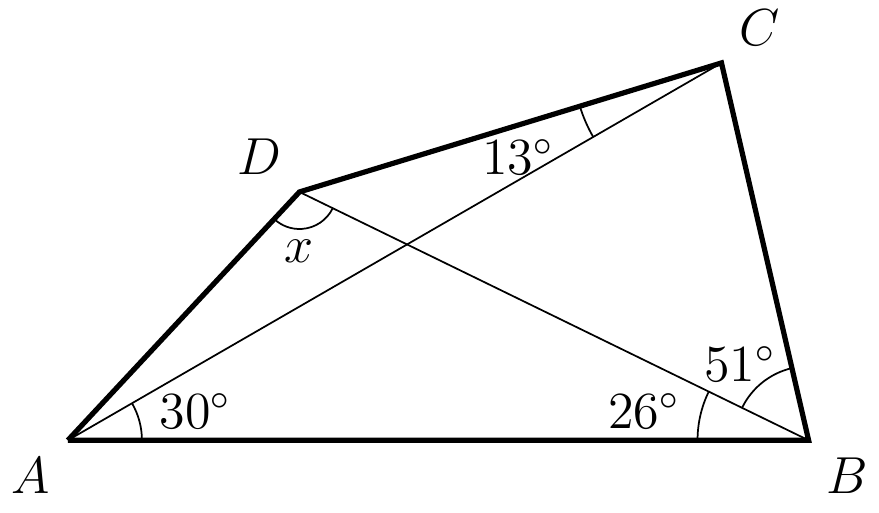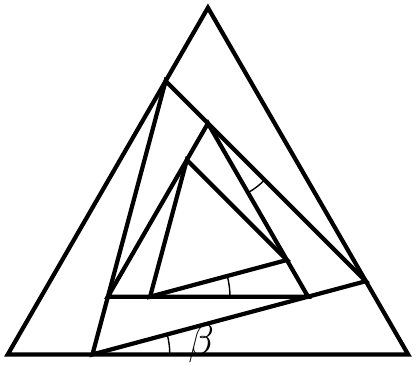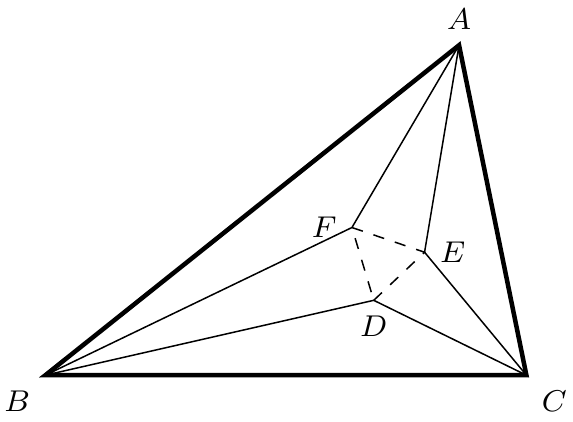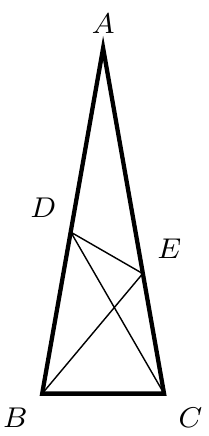###### back to index | new

Find the least non-negative residue of $70! \pmod{5183}$.

Show that it is impossible to cover an $8\times 8$ square using fifteen $4\times 1$ rectangles and one $2\times 2$ square.

It is possible to cover a $6\times 6$ grid using one L-shaped piece made of 3 grids and eleven $3\times 1$ smaller grid ?

Two players, $A$ and $B$, take turns naming positive integers, with $A$ playing first. No player may name an integer that can be expressed as a linear combination, with positive integer coefficients, of previously named integers. The player who names 1 loses. Show that no matter how A and B play, the game will always end.

$\textbf{Heaps of Beans}$

A game starts with four heaps of beans, containing $3$, $4$, $5$ and $6$ beans, respectively. The two players move alternately. A move consists of taking either one bean from a heap, provided at least two beans are left behind in that heap, or a complete heap of two or three beans. The player who takes the last bean wins. Does the first or second player have a winning strategy?

A positive integer $n$ is said to be good if there exists a perfect square whose sum of digits in base $10$ is equal to $n$. For instance, $13$ is good because $7^2 = 49$ and $4 + 9 = 13$. How many good numbers are among $1, 2, 3, \cdots , 2007$?

Prove that the sum $$\sqrt{1001^2 + 1} + \sqrt{1002^2 + 1} + \cdots + \sqrt{2000^2 + 1}$$ is irrational.

Let $n$ be an even positive integer, and let $p(x)$ be an $n$-degree polynomial such that $p(-k) = p(k)$ for $k = 1, 2, \dots , n$. Prove that there is a polynomial $q(x)$ such that $p(x) = q(x^2)$.

Let $a, b, c$ be distinct integers. Can the polynomial $(x - a)(x - b)(x - c) - 1$ be factored into the product of two polynomials with integer coefficients?

Let $P(x) = x^n + a_{n-1}x^{n-1} + \cdots + a_1x + a_0$ be a polynomial with integral coefficients. Suppose that there exist four distinct integers $a, b, c, d$ with $P(a) = P(b) = P(c) = P(d) = 5$. Prove that there is no integer $k$ satisfying $P(k) = 8$.

Show that $(1 + x + \cdots + x^n)^2 - x^n$ is the product of two polynomials.

Determine all polynomials such that $P(0) = 0$ and $P(x^2 + 1) = P(x)^2 + 1$.

Find the largest integer not exceeding $1 + \frac{1}{\sqrt{2}}+ \frac{1}{\sqrt{3}} + \cdots + + \frac{1}{\sqrt{10000}}$

Let $n$ be an integer greater than or equal to 3. Prove that there is a set of $n$ points in the plane such that the distance between any two points is irrational and each set of three points determines a non-degenerate triangle with rational area.

A highly valued secretive recipe is kept in a safe with multiple locks. Keys to these locks are distributed among 9 members of the managing committee. The established rule requires that at least 6 members must present in order to open the safe. What is the minimal number of locks required? Correspondingly, how many keys are required?

Let $a_n=\binom{200}{n}(\sqrt{6})^{200-n}\left(\frac{1}{\sqrt{2}}\right)^n$, where $n=1$, $2$, $\cdots$, $95$. Find the number of integer terms in $\{a_n\}$.

Let sequence $\{a_n\}$ satisfy the condition: $a_1=\frac{\pi}{6}$ and $a_{n+1}=\arctan(\sec a_n)$, where $n\in Z^+$. There exists a positive integer $m$ such that $\sin{a_1}\cdot\sin{a_2}\cdots\sin{a_m}=\frac{1}{100}$. Find $m$.

As shown in diagram below, find the degree measure of $\angle{ADB}$.An infinite number of equilateral triangles are constructed as shown on the right. Each inner triangle is inscribed in its immediate outsider and is shifted by a constant angle $\beta$. If the area of the biggest triangle equals to the sum of areas of all the other triangles, find the value of $\beta$ in terms of degrees.For $-1 < r < 1$, let $S(r)$ denote the sum of the geometric series $$12+12r+12r^2+12r^3+\cdots .$$Let $a$ between $-1$ and $1$ satisfy $S(a)S(-a)=2016$. Find $S(a)+S(-a)$.

Find the least positive integer $m$ such that $m^2 - m + 11$ is a product of at least four not necessarily distinct primes.

(Weitzenbock's Inequality) Let $a, b, c$, and $S$ be a triangle's three sides' lengths and its area, respectively. Show that $$a^2 + b^2 + c^2 \ge 4\sqrt{3}\cdot S$$

Prove that there is one and only one triangle whose side lengths are consecutive integers, and one of whose angles is twice as large as another.

In $\triangle{ABC}$, $AE$ and $AF$ trisects $\angle{A}$, $BF$ and $BD$ trisects $\angle{B}$, $CD$ and $CE$ trisects $\angle{C}$. Show that $\triangle{DEF}$ is equilateral.As shown, in $\triangle{ABC}$, $AB=AC$, $\angle{A} = 20^\circ$, $\angle{ABE} = 30^\circ$, and $\angle{ACD}=20^\circ$. Find the measurement of $\angle{CDE}$.